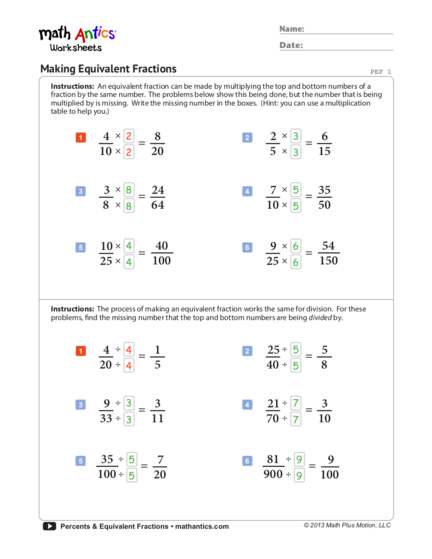# Equivalent Fractions by Multiplication and DivisionContributed by:Equivalent fractions by Multiplication and Division worksheet. Equivalent fractions are the fractions that have different numerators and denominators but are equal to the same value. For example, 2/4 and 3/6 are equivalent fractions, because they both are equal to the ½. A fraction is a part of a whole. Equivalent fractions represent the same proportion of the whole.
1. ® Name:
Date:
Making Equivalent Fractions PEF 1
Instructions: An equivalent fraction can be made by multiplying the top and bottom numbers of a
fraction by the same number. The problems below show this being done, but the number that is being
multiplied by is missing. Write the missing number in the boxes. (Hint: you can use a multiplication
1 4 × 2
= 8 2 2 × 3
= 6
10 × 2 20 5 × 3 15
3 3 × 8
= 24 4 7 × 5
= 35
8 × 8 64 10 × 5 50
5 10 × 4 40 6 9 × 6 54
= =
25 × 4 100 25 × 6 150
Instructions: The process of making an equivalent fraction works the same for division. For these
problems, find the missing number that the top and bottom numbers are being divided by.
1 4 ÷ 4
= 1 2 25 ÷ 5
= 5
20 ÷ 4 5 40 ÷ 5 8
3 9 ÷ 3
= 3 4 21 ÷ 7
= 3
33 ÷ 3 11 70 ÷ 7 10
35 ÷ 81 ÷
= 7 = 9
5 5 6 9
100 ÷ 5 20 900 ÷ 9 100
Percents & Equivalent Fractions • mathantics.com © 2013 Math Plus Motion, LLC
2. ® Name:
Date:
Equivalent Percent Form PEF 2
Instructions: Convert each of these fractions into an equivalent fraction that has 100 as the
bottom number. Then write that fraction in its percent form. (Some will need to be converted
by multiplying and others by dividing.)
1 3 ×5 15 2 1 ×5 5
= = 15% = = 5%
20 ×5 100 20 ×5 100
3 12 ×2 24 4 80 ÷ 2 40
= = 24% = = 40%
50 ×2 100 200 ÷ 2 100
5 8 ×4 32 6 8 × 10 80
= = 32% = = 80%
25 ×4 100 10 × 10 100
7 16 ÷ 4 4 8 24 ÷ 3 8
= = 4% = = 8%
400 ÷ 4 100 300 ÷ 3 100
9 3 × 20 60 10 3 × 50 150
= = 60% = = 150%
5 × 20 100 2 × 50 100
11 45 ÷ 5 9 12 30 ×4 120
= = 9% = = 120%
500 ÷ 5 100 25 ×4 100
Percents & Equivalent Fractions • mathantics.com © 2013 Math Plus Motion, LLC
3. ® Name:
Date:
Equivalent Percent Form - Set 2 PEF 3
Instructions: Convert each of these fractions into an equivalent fraction that has 100 as the
bottom number. Then write that fraction in its percent form. (Some will need to be converted
by multiplying and others by dividing.)
1 50 ÷ 2 25 2 7 × 10 70
= = 25% = = 70%
200 ÷ 2 100 10 × 10 100
3 10 ÷ 5 2 4 32 ÷ 4 8
= = 2% = = 8%
500 ÷ 5 100 400 ÷ 4 100
5 11 ×4 44 6 400 ÷ 8 50
= = 44% = = 50%
25 ×4 100 800 ÷ 8 100
7 49 ÷ 7 7 8 7 × 20 140
= = 7% = = 140%
700 ÷ 7 100 5 × 20 100
9 15 ×2 30 10 3 × 50 150
= = 30% = = 150%
50 ×2 100 2 × 50 100
11 7 ×5 35 12 60 ÷ 6 10
= = 35% = = 10%
20 ×5 100 600 ÷ 6 100
Percents & Equivalent Fractions • mathantics.com © 2013 Math Plus Motion, LLC
4. ® Name:
Date:
Equivalent Fractions: Unknown Top Number PEF 4
Instructions: In each pair of equivalent fractions below, a top number is unknown. Figure out
what the relationship between the bottom numbers is. (In other words, figure out what was
multiplied or divided by to get the new bottom number.) Then do that same thing to the top
number to find the unknown value (n).
so to find ‘n’, we also
×6 multiply by 6.
Example 2 = n
5 30 n=2×6
×6
n = 12
to go from 5 to 30, we
have to multiply by 6
×2 ×4
1 4 = n n=4×2 2 4 = n n=4×4
10 20 n=8 6 24 n = 16
×2 ×4
÷ 10 ×7
3 30 n n = 30 ÷ 10 4 1 n n=1×7
= =
50 5 n=3 3 21 n=7
÷ 10 ×7
×3 ÷8
5 9 = n n=9×3 6 24 = n n = 24 ÷ 8
20 60 n = 27 64 8 n=3
×3 ÷8
×4 ×6
7 12 = n n = 12 × 4 8 3 = n n=3×6
25 100 n = 48 11 66 n = 18
×4 ×6
÷3 ×5
9 21 = n n = 21 ÷ 3 10 12 = n n = 12 × 5
150 50 n=7 8 40 n = 60
÷3 ×5
Percents & Equivalent Fractions • mathantics.com © 2013 Math Plus Motion, LLC